# 33 Practice Exercises

Exercise: Phase Change – Carbon Dioxide

Identify the phase changes that carbon dioxide will undergo as its temperature is increased from −100 °C while holding its pressure constant at 1,000 kPa. At what approximate temperatures do these phase changes occur? The phase diagram of carbon dioxide is shown below.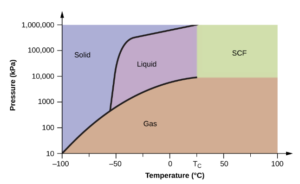Image obtained from OpenStax Chemistry/ CC BY 4.0

Note: This diagram is missing the “vapour” phase. Usually, we call the gaseous phase under the liquid region “vapor” because it is able to condense to a liquid, while the “gas” phase is in a temperature that is too high to condense. Sometimes the terms are not strictly distinguished.

### Solution

The phase change from solid to liquid at ~-55°C; from liquid to gas at ~-40°C.

To represent holding pressure constant while increasing temperature, we can draw a line moving horizontally to the right on the phase diagram.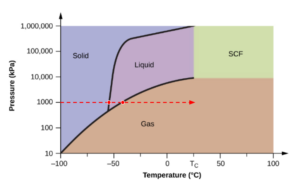A phase change occurs every time when the horizontal line hits a curve on the diagram.

Exercise: Gibb’s Phase Rule

How many degrees of freedom exist for a vapour-liquid (V-L) mixture of acetone ($(CH_3)_2CO$) and methyl ethyl ketone ($CH_3C(O)CH_2CH_3$)? Assume no chemical reaction happens.

### Solution

vapour-liquid mixture: $\pi=2$

mixture of acetone and methyl ethyl ketone: $c=2$

no chemical reactions: $r=0$

$$D\!F=2+c-\pi-r=2+2-2-0=2$$

Exercise: Ideal Gas Law

Calculate the density (mass per cubic metre) of dry air (a) under IUPAC standard conditions and (b) in a hot air balloon at a temperature of 120°C under the same pressure. Assuming ideal gas behaviour.$^{}$

Take average molar mass =29.0 mol/L for dry air. $R=8.314\frac{J}{molK}$

### Solution

a) To find the mass per cubic metre, we need the number of moles contained in a cubic metre under the IUPAC standard temperature and pressure. Calculate n by rearranging the ideal gas law:
\begin{align*}
PV & = nRT \\
n & =\frac{PV}{RT}\\
n & =\frac{1×10^5Pa×1m^3}{8.314\frac{J}{molK}×273.15K}\\
n & = 44.1mol
\end{align*}

The mass of air is equal to the number of moles contained in a cubic metre multiplied by molar mass:
$$m=n×MW=44.1mol×29.0g/mol=1278.9g=1.28kg$$

The density of air is the mass divide by volume:
$$\rho=\frac{m}{V}=\frac{1.28kg}{1m^3}=1.28kg/m^3$$

b) Here, we also have the same volume (1 $m^3$) but different temperature. We can once again find the number of moles this will hold.
\begin{align*}
PV & = nRT \\
n & =\frac{PV}{RT}\\
n & =\frac{1×10^5Pa×1m^3}{8.314\frac{J}{molK}×(273.15+120)K}\\
n & = 30.6mol
\end{align*}

We do a similar calculation as before to find the mass of the gas in the cubic metre
$$m=n×MW=30.6mol×29.0g/mol=887.4g=0.89kg$$

We can then use this to find density under this new condition:
$$\rho=\frac{m}{V}=\frac{0.89kg}{1m^3}=0.89kg/m^3$$

This is less dense, which makes sense as the temperature is higher, so we would expect less gas molecules in the same volume at a similar pressure.

One other way to solve this is to observe that the density is proportional to the number of moles, which is inversely proportional to the temperature:
\begin{align*}
\frac{\rho_{2}}{\rho_{1}} & = \frac{T_{1}}{T_{2}} \\
\rho_{2} & =\frac{T_{1}}{T_{2}}\rho_{1}\\
\rho_{2} & =\frac{273.15K}{(120+273.15)K}×1.28kg/m^3\\
\rho_{2} & = 0.89 kg/m^3
\end{align*}

Exercise: Manometer in a Pressure Alarm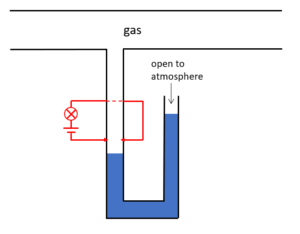a) The figure demonstrates the levels of the liquid at normal conditions. Is the pressure of the gas in the sealed tube higher or lower than atmospheric pressure?

b) We use a circuit as shown by the red lines to report abnormal pressure in the tube. The liquid in the manometer acts as a switch: when a conductor is placed in between the red arrows, the circuit will be closed and the light bulb will be turned on.
Suppose the gas in the tube is an insulator and the liquid is a conductor, the light turns on means: ______ (choose from: the tube leaks or depressurizes/ the gas in the tube is over-pressurized).

### Solution

a) The pressure of the gas in the sealed tube is higher than atmospheric pressure.

b) The tube leaks or depressurizes.

Closing the circuit requires the liquid level to rise up to the level of the red arrows. When the pressure in the gas tube decreases, the liquid level on the left side will increase to balance the pressure.

Exercise: Measurement Range of a Pressure Gauge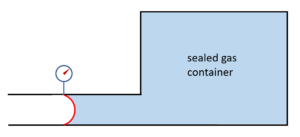Suppose we use a flexible membrane to measure the pressure in a sealed container. The membrane is attached to the atmosphere on one side (take$P_{atm} = 1.0×10^5 Pa$) and a sealed container of gas at the other. The membrane has an area of 0.005$m^2$ and will be permanently deformed when experiencing a net force larger than 300N.

a) It is known that the absolute pressure in the gas container is 89kPa at 273K temperature. What is the range of pressure in the container that can be measured without permanently deforming the membrane?

b) Assuming the volume of the container is constant, what is the corresponding temperature range that allows the pressure in the container to be measured?

### Solution

a) The net force experienced by the membrane is caused by the difference of the pressure in the gas container and atmospheric pressure (gauge pressure). Using the definition of pressure $P=\frac{F}{A}$, we can calculate the range of gauge pressure we can measure with the membrane. The net force can be exerted in both directions, which means the gauge pressure may be positive to negative:
$$P=\frac{F}{A}=\frac{300N}{0.005m^2}=60000Pa$$
So the range of gauge pressure we can measure is: $$-60000Pa<P<60000Pa$$
The range of absolute pressure we can measure is:
\begin{align*}
1.0×10^5Pa-60000Pa<&P<1.0×10^5Pa+60000Pa\\
0.4×10^5Pa<&P<1.6×10^5Pa
\end{align*}

b) We can calculate the corresponding temperatures by rearranging the ideal gas law:
$$n=\frac{P_1V_1}{RT_1}=\frac{P_2V_2}{RT_2}$$
Because R and the volume of the container are constant, we can simplify the equation:
$$\frac{P_1}{T_1}=\frac{P_2}{T_2}$$
And we can solve for the temperature range by substituting in the range of absolute pressure and the known set of temperature and pressure:
\begin{align*}
\frac{1.6×10^5Pa}{T_{high}}&=\frac{89×10^3Pa}{273K}\\
T_{high}&=\frac{1.6×10^5Pa×273K}{89×10^3Pa}\\
&=491K
\end{align*}
\begin{align*}
\frac{0.4×10^5Pa}{T_{low}}&=\frac{89×10^3Pa}{273K}\\
T_{low}&=\frac{0.4×10^5Pa×273K}{89×10^3Pa}\\
&=123K
\end{align*}
So the temperature range to measure the pressure in the container is $$123K<T<491K$$

Exercise: Pxy Diagram

The following graph shows the Pxy diagram for a mixture of n-hexane and n-heptane at a constant temperature of 373K.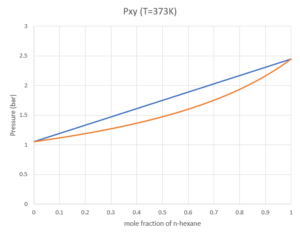Using the diagram above, answer the following questions:

a) What is the vapour pressure of pure n-hexane at T=373K?

b) What is the phase(s) in the system when the mole fraction of n-hexane in the system is 0.3 at a pressure of 2 bar?

c) What is the dew point pressure for a mixture with 30% n-heptane?

d) At vapour-liquid equilibrium, if the mole fraction of n-hexane in the liquid phase is 0.3, what is the mole fraction of n-hexane in the vapour phase?

e) At vapour-liquid equilibrium, if the mole fraction of n-hexane in the vapour phase is 0.3, what is the mole fraction of n-hexane in the liquid phase?

### Solution

(Since the answers are read from the graph, each question will allow a range of correct answers)

a) 2.45 bar
Find the y-coordinate for the point when the mole fraction of n-hexane is 1 and the two lines meet at a point.

b)the system only has a liquid phase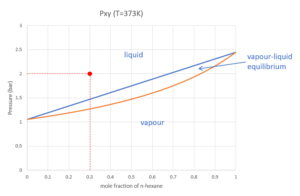c) 1.75 bar
Because the x-axis represents the mole fraction of n-hexane, we need to convert 30% n-heptane to mole fraction of n-hexane=0.7: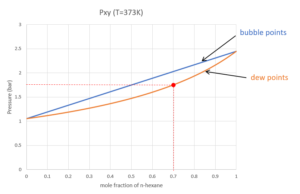d) $y_{n-hexane}=0.5$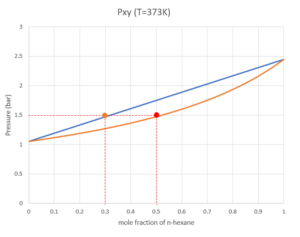e) $x_{n-hexane}=0.5$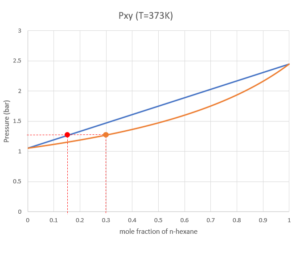Exercise: Txy Diagram

The following graph shows the Txy diagram for a mixture of n-hexane and n-heptane at a constant pressure of 1.013 bar.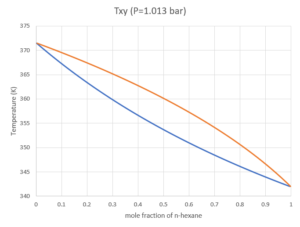Using the diagram above, answer the following questions:

a) What is the boiling temperature for pure n-heptane at P=1.013 bar?

b) What is the bubble point temperature when the mole fraction of n-hexane is 0.6?

c) If the mole fraction of n-hexane is 0.4, at T=360K, what is the mole fraction of n-hexane in liquid and vapour phase?

d) Say we use the following process to purify the n-hexane in the stream:

• We start with a liquid mixture of  50% n-hexane at its bubble point
• The liquid mixture is sent to an isothermal distillation column, where the system is kept at a steady-state of vapour-liquid equilibrium at the bubble point, and we collect the vapour into the output stream as soon as the vapour is formed
• The vapour is cooled to the bubble point
• We send the liquid into another isothermal distillation column, where we collect the vapour formed at the bubble point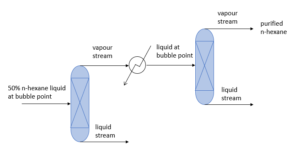What is the mole fraction of n-hexane in the purified stream?

### Solution

(Because the answer is read from the graph, each question will allow a range of correct answers)

a)372K
Find the y-coordinate for the point when the mole fraction of n-hexane is 0 and the two lines meet at a point.

b) 351K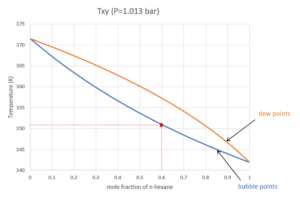c) $x_{n-hexane}=0.3$, $y_{n-hexane}=0.5$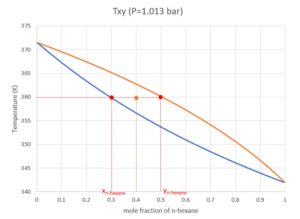d) mole fraction of n-hexane in the purified stream=0.85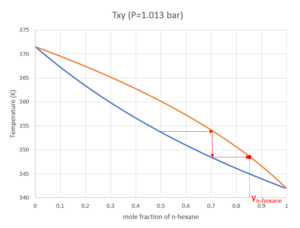## References

 OpenStax University Physics Volume 2. 2016. 2.1 Molecular Model of an Ideal Gas. [online] <https://openstax.org/books/university-physics-volume-2/pages/2-1-molecular-model-of-an-ideal-gas> [Accessed 14 May 2020].